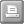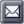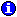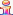﻿ In-line Math and Date Math
Pathagoras Help System

# In-line Math and Date Math# In-line Math and Date MathAs the previous sections indicate, Pathagoras has the ability to perform simple mathematical calculations and concatenations of 'text'. While pretty 'good' for a plain-text based program, it still required that the user create a mask to store the formula, making the documents a little less universal.

Masks are actually wonderful things, but the reality is that they are more challenging to create (or to remember to create).

Beginning with version 2012.2, Pathagoras offers the user to ability to insert math and concatenation formulas 'in-line' with the source text (as opposed to requiring the formula to be saved in a mask).

A precise structure is required (examples shown below), but its really not that hard:

The 'formula' is:

1. square brackets surrounding the entire variable term;

2. the call-to-action "#Math#" (no quotes) placed immediately after the opening bracket;

3. the variable name;

4. a equal sign to indicate the equivalency;

5. the formula, which is simply a reference to the existing variable the math you wish to perform. Note that there will always be a nesting of the variables, and the closing of the variable will very frequently be a double ']]'.

Here are some examples. The first elements are simply plain variables. They need to be completed in order for any in-line math to be performed. There is no order requirement EXCEPT that all references must be on the same Instant Database page as the formula.

Simple Math:

[Quantity]

[Price]

[#Math#total price=[Quantity]x[Price]]

[#Math#half unit price=[Price]/2]

[#Math#half total price=[Quantity]x[Price]/2]

(Note: multiplication must be indicated by 'x', not '*')

Date Math ('termination date' is the target variable):

This contract will begin on [start date].

It will automatically renew on [#Math#termination date=[start date] + [3 months]], and every 3 months thereafter.

If you want to return the 'expiry' date (the day before the 'typical' return date, use this setup:

This contract will begin on [start date].

It will expire at midnight on [#Math#termination date=[start date] + [3 months(exp)]].

Age Math ('Age of H' is the target variable):

Husband was born on [Birthday of H].

He is [#Math#Age of H=[Today]-[Birthday of H]] years old.

(Note: When the variable ]Today] is in the formula, you need not pre-set its value.One might say that the above formulas are pretty complex to set up. While it may be true, keep this in mind. Math in any document assembly program requires setting up a formula. With Pathagoras, the formula is shown in-line and on the face of the document. That makes it more accessible for editing, and more accessible just for 'seeing' what is happening.Once the variable in the in-line formula has been 'calculated,' the variable can be used in other parts of the document without the 'formula'. So once 'total price' or 'half unit price' or 'termination date', etc. have been used and processed in a document, that variable can be referenced later in the document as a 'normal' variable, e.g.,  [total price] or [half unit price] or [termination date]. After all, it's just a plain text variable!By default, Pathagoras will return the calculated value in number of days. To return weeks, months or years, be sure to include the term 'weeks' 'months' or 'years' somewhere in the formula. E.g.,

John Smith worked for Spacely Sprockets for [#Math#duration in weeks=[Client 1 Empl Start Date] -[Client 1 Empl End Date]] weeks.